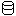# Code Smells

Code Smells / Dispensables / Data Class# Data Class

A Data Class smell occurs when a class does not implement enough functionality itself to justify it being a class.

This means that the class defines instance variables, but lacks relevant methods. Such classes are very likely being manipulated by other classes heavily.

``````from math import sqrt

# Smelly code
class Coordinates:
def __init__(self, x_coordinate, y_coordinate, z_coordinate):
self.x_coordinate = x_coordinate
self.y_coordinate = y_coordinate
self.z_coordinate = z_coordinate

my_coordinates = Coordinates(4, -7, 43)
print(sqrt(my_coordinates.x_coordinate**2 + my_coordinates.y_coordinate**2 + my_coordinates.z_coordinate**2))  # Pythagorean theorem

# Fixed code
class Coordinates:
def __init__(self, x_coordinate, y_coordinate, z_coordinate):
self.x_coordinate = x_coordinate
self.y_coordinate = y_coordinate
self.z_coordinate = z_coordinate

def distance(self):
return sqrt(self.x_coordinate**2 + self.y_coordinate**2 + self.z_coordinate**2)  # Pythagorean theorem

my_coordinates = Coordinates(4, -7, 43)
print(my_coordinates.distance())
``````

Often, this is an indication that relevant functionality for the data that it stores is being implemented elsewhere in the code, or that the data stored in objects of the class should be stored in another class.

To avoid this code smell, ensure that relevant functionality for the data is implemented in methods in the class, and that the data is being stored in the class which is most appropriate for it.